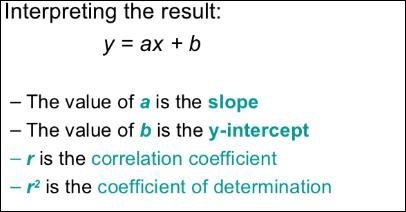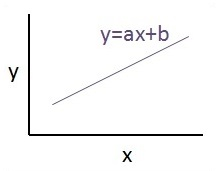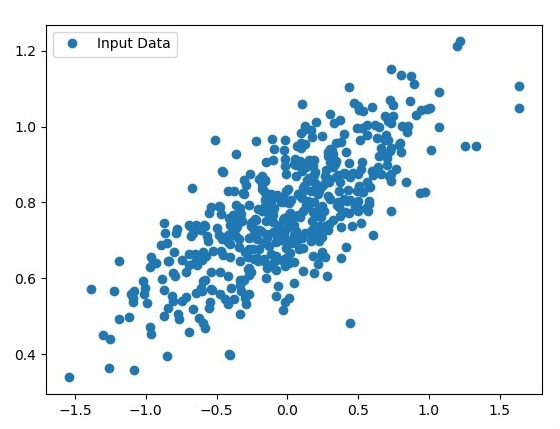# TensorFlow - Linear Regression

In this chapter, we will focus on the basic example of linear regression implementation using TensorFlow. Logistic regression or linear regression is a supervised machine learning approach for the classification of order discrete categories. Our goal in this chapter is to build a model by which a user can predict the relationship between predictor variables and one or more independent variables.

The relationship between these two variables is cons −idered linear. If y is the dependent variable and x is considered as the independent variable, then the linear regression relationship of two variables will look like the following equation −

Y = Ax+b


We will design an algorithm for linear regression. This will allow us to understand the following two important concepts −

• Cost Function

The schematic representation of linear regression is mentioned below −The graphical view of the equation of linear regression is mentioned below −## Steps to design an algorithm for linear regression

We will now learn about the steps that help in designing an algorithm for linear regression.

### Step 1

It is important to import the necessary modules for plotting the linear regression module. We start importing the Python library NumPy and Matplotlib.

import numpy as np
import matplotlib.pyplot as plt


### Step 2

Define the number of coefficients necessary for logistic regression.

number_of_points = 500
x_point = []
y_point = []
a = 0.22
b = 0.78


### Step 3

Iterate the variables for generating 300 random points around the regression equation −

Y = 0.22x+0.78

for i in range(number_of_points):
x = np.random.normal(0.0,0.5)
y = a*x + b +np.random.normal(0.0,0.1) x_point.append([x])
y_point.append([y])


### Step 4

View the generated points using Matplotlib.

fplt.plot(x_point,y_point, 'o', label = 'Input Data') plt.legend() plt.show()


The complete code for logistic regression is as follows −

import numpy as np
import matplotlib.pyplot as plt

number_of_points = 500
x_point = []
y_point = []
a = 0.22
b = 0.78

for i in range(number_of_points):
x = np.random.normal(0.0,0.5)
y = a*x + b +np.random.normal(0.0,0.1) x_point.append([x])
y_point.append([y])

plt.plot(x_point,y_point, 'o', label = 'Input Data') plt.legend()
plt.show()


The number of points which is taken as input is considered as input data.